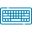# Difference Quotient Calculator## Table of Content

Feedback

Our Difference Quotient Calculator is used to approximate the derivative of a function at a given point by finding the rate of change or slope of a function.  It calculates how much the function's value changes when you make a small adjustment to the input value. This can be useful in mathematical analysis and scientific applications.

## Steps to Use Difference Quotient Calculator:

No matter if you are a student studying calculus or a professional analyzing trends, this calculator simplifies complex calculations. Below you will find some simple steps that show how to use the Difference Quotient Calculator effectively.

What to Enter?

• Step 1: First, Choose a variable from the drop-down list
• Step 2: Then, Enter a function (f) into the field
• Step 3: Press the Calculate button

What will you get?

• Difference quotients for the given function
• Step-by-step calculations

## Difference Quotient Formula:

The Difference Quotient Formula is a mathematical expression used to find the average rate of change of a function between two specific points on its graph. The equation to find the difference quotient is as follows:

$$\Large \frac{f(x+h)-f(x)}{h}$$

Where,

• f(x) – The value of a function at a specific input “x”
• f(x+h) – The value of the same function at a slightly different input (x + h)
• h – A small constant representing the change in the input.

You can easily calculate the average rate of change of the function f(x) over a small interval
[x, x + h] by using this formula.

## How to Find the Difference Quotient?

In this section, we’ll learn how to calculate the difference quotient manually by illustrating an example so that you can easily understand the concepts better. You can also try our difference quotient calculator for this purpose.

### Example:

Suppose we have a function $f(x) = 3x^2 - 2x + 1$, and we want to find the average rate of change of this function between two points, x = 2 and x = 4. This means we want to calculate the slope of the secant line that passes through these two points from x = 2 to x = 4.

### Solution:

1. First, we need to calculate the change in x. In this case, h = 4 - 2 = 2. This represents the difference between our two points.

2. Now, let's apply the Difference Quotient formula:

$$\text{Difference Quotient} = \frac{f(x + h) - f(x)}{h}$$

3. Put in all the values:

\begin{align*} \text{Difference Quotient} &= \frac{f(4) - f(2)}{2} \\ &= \frac{(3(4)^2 - 2(4) + 1) - (3(2)^2 - 2(2) + 1)}{2} \end{align*}

4. Calculate the values inside the parentheses:

\begin{align*} \text{Difference Quotient} &= \frac{(3(16) - 8 + 1) - (3(4) - 4 + 1)}{2} \\ &= \frac{(48 - 8 + 1) - (12 - 4 + 1)}{2} \end{align*}

5. Simplify the difference quotient further:

\begin{align*} \text{Difference Quotient} &= \frac{(41) - (9)}{2} \\ &= \frac{32}{2} \\ &= 16 \end{align*}

So, the average rate of change of the function f(x) = 3x^2 - 2x + 1 between x = 2 and x = 4 is 16.

## FAQs:

### What is the Difference Quotient?

In Mathematics, the Difference Quotient is used to calculate the average rate of change of a function or the slope of a curve over a short interval. Basically, it measures how a function's output (or dependent variable) changes with a small change in its input (or independent variable).

### What is the limit of a difference quotient?

The limit of a difference quotient is like a way to find the exact rate of change of a function at a specific point. Imagine zooming in so closely on a curve that it looks almost straight at that point. The limit tells you the slope of that tiny, straight line. It's like finding the true speed of something at a single moment, not just an average speed over a distance.

## References:

Storyofmathematics.com: Difference Quotient, What is the difference quotient? Understanding the difference quotient formula, How to evaluate difference quotient? and How to simplify difference quotient?### Alan Walker

Studies mathematics sciences, and Technology. Tech geek and a content writer. Wikipedia addict who wants to know everything. Loves traveling, nature, reading. Math and Technology have done their part, and now it's the time for us to get benefits.